Updating search results...

# 15 Results

View
Selected filters:
• 3rd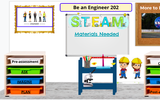Unrestricted Use
CC BY
Rating
0.0 stars
Overview:

Students discover engineering and use the design thinking process to create prototypes to show their learning about engineering.

Subject:
Engineering
Technology Engineering and Design Education
STEM
Material Type:
Activity/Lab
Module
Self Assessment
Author:
Becky (REBECCA) SNYDER
05/27/2022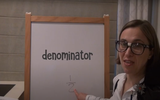Unrestricted Use
CC BY
Rating
0.0 stars
Overview:

This lesson is Day 10 in a series of 12 lessons around fraction equivalences and comparisons.

This video lesson serves as a review of equpartitioning in order to determine the fractional part of an area or length. This is a necessary foundational skill for comparing and finding equivalent fractions.

Subject:
Mathematics
Material Type:
Activity/Lab
Interactive
Lesson
Simulation
Author:
Dawne Coker
06/25/2020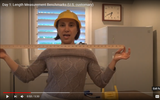Unrestricted Use
CC BY
Rating
0.0 stars
Overview:

This lesson is Day 1 in a series of 5 lessons around U.S customary measurement.

This 3rd grade video lesson introduces the U.S. customary measurements for length: inches, feet, and yards. In this lesson, students will learn benchmarks (visuals) for measuring in inches, feet and yards when they don't have standard measurement tools available.

Subject:
Mathematics
Material Type:
Activity/Lab
Interactive
Lesson
Simulation
Author:
Dawne Coker
06/25/2020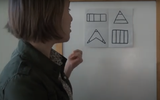Unrestricted Use
Public Domain
Rating
0.0 stars
Overview:

This lesson is Day 1 in a series of 12 lessons around fraction equivalences and comparisons.

This video lesson encourages students to reason about and visualize fractions. The lesson focuses on the ideas that fractions are fair shares and that fractions can be compared. This lesson assumes that students have some initial experiences with fractions.

Subject:
Mathematics
Material Type:
Interactive
Lesson
Presentation
Simulation
Author:
Dawne Coker
06/25/2020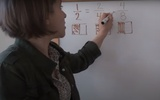Unrestricted Use
Public Domain
Rating
0.0 stars
Overview:

This lesson is Day 2 in a series of 12 lessons around fraction equivalences and comparisons.

This video lesson uses paper folding to introduce the concept of equivalent fractions. To complete this lesson, make sure you have a square piece of paper (any size) and a crayon

Subject:
Mathematics
Material Type:
Activity/Lab
Interactive
Lesson
Simulation
Author:
Dawne Coker
06/25/2020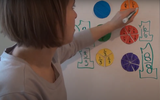Unrestricted Use
CC BY
Rating
0.0 stars
Overview:

This lesson is Day 2 in a series of 12 lessons around fraction equivalences and comparisons.

This video lesson introduces the idea that fractions can be used to represent 1 whole. To engage in this lesson, students may use virtual circle models from Toy Theater (https://toytheater.com/fraction-circles/)

Subject:
Mathematics
Material Type:
Activity/Lab
Interactive
Lesson
Simulation
Author:
Dawne Coker
06/25/2020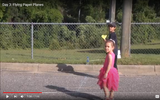Unrestricted Use
CC BY
Rating
0.0 stars
Overview:

This lesson is Day 3 in a series of 5 lessons around U.S customary measurement.

This video lesson explores the use of appropriate units for measuring length. Additionally, students will be asked to solve single-step problems involving lengths of distances. No materials are needed for this lesson.

Subject:
Mathematics
Material Type:
Activity/Lab
Interactive
Lesson
Simulation
Author:
Dawne Coker
06/25/2020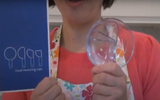Unrestricted Use
CC BY
Rating
0.0 stars
Overview:

This lesson is Day 4 in a series of 12 lessons around fraction equivalences and comparisons.

This video lesson looks at how we use fractions in the kitchen. Students are challenged to use circle models to find equivalent fractions for a recipe. Students may use virtual circe models from the Toy Theater (https://toytheater.com/fraction-circles/).

Subject:
Mathematics
Material Type:
Activity/Lab
Interactive
Lesson
Simulation
Author:
Dawne Coker
06/25/2020Unrestricted Use
CC BY
Rating
0.0 stars
Overview:

This lesson is Day 5 in a series of 5 lessons around U.S customary measurement.

In this video lesson, students learn the difference between weight and capacity as they observe a cookie recipe being made. No materials are needed for this lesson.

Subject:
Mathematics
Material Type:
Activity/Lab
Interactive
Lesson
Simulation
Author:
Dawne Coker
06/25/2020Unrestricted Use
CC BY
Rating
0.0 stars
Overview:

This lesson is Day 6 in a series of 12 lessons around fraction equivalences and comparisons.

This video lesson teaches students how to create bar models to compare lengths/distances. Students will need a piece of paper, ruler, scissors, and a pencil. These bar models will also be used for Day 7's lesson.

Subject:
Mathematics
Material Type:
Activity/Lab
Interactive
Lesson
Simulation
Author:
Dawne Coker
06/25/2020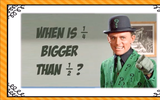Unrestricted Use
CC BY
Rating
0.0 stars
Overview:

This video lesson introduces the idea that when comparing two fractions, they must refer to the same size whole. Then, students use fraction strips to compare fractions. Students may use virtual fraction strips from Toy Theater (https://toytheater.com/fraction-strips/)

Subject:
Mathematics
Material Type:
Activity/Lab
Interactive
Lesson
Simulation
Author:
Dawne Coker
06/25/2020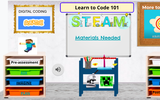Unrestricted Use
CC BY
Rating
0.0 stars
Overview:

Explore what coding is and create your own game without using a computer!

Subject:
Computer Science
Engineering
Information and Technology
Technology Engineering and Design Education
STEM
Material Type:
Activity/Lab
Lesson Plan
Module
Self Assessment
Student Guide
Author:
Becky (REBECCA) SNYDER
05/27/2022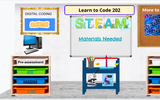Unrestricted Use
CC BY
Rating
0.0 stars
Overview:

Investigate what coding is and use the design thinking process to create your own game, without a computer!

Subject:
Computer Science
Engineering
STEM
Material Type:
Activity/Lab
Module
Self Assessment
Student Guide
Author:
Becky (REBECCA) SNYDER
05/27/2022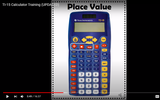Unrestricted Use
CC BY
Rating
0.0 stars
Overview:

This video demonstrates the functions of the TI-15 calculator. It may be used as a professional development for teachers, or for training students. It is not recommended that the video is shown to students in its entirety. If you scroll down to the comments section under the video, time markers for each function are provided. Click on the time markers to go directly to that specific function.

Subject:
Mathematics
Material Type:
Activity/Lab
Diagram/Illustration
Interactive
Teaching/Learning Strategy
Author:
Dawne Coker